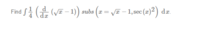# Integration by Substitution

#### AnnaGautrey

##### New member
I have a question strangely worded and I don't have the solution so I wanted to make sure I was doing it rightI know I am supposed to use the inverse chain rule to solve this.
I have subbed in sqrt(x)-1 into the x already inside the integral, then subbed the x into that new function to get

integral ( 1/4 * d/dx( sqrt(sec(x) -1))

then solved for the derivative to get

integral ( 1/4 * ( 1/2*(sec(x)-1)^-(1/2) * sec(x)tan(x) ) )

so then used the inverse chain rule to get

1/4 * (sec(x)-1)^(1/2) + c

would this be correct?

#### Jomo

##### Elite Member
Take the derivative of your answer and see if you get back the integrand.

Suppose d/dx (f(x)) = F(x). So the integral(F(x))dx = f(x).
Do you see this?
Then what is the answer to your question?

#### AnnaGautrey

##### New member
if you sub the original x values back in you would get 1/4*sqrt(x) + c
if you did the question without substitution you would just get 1/4*sqrt(x) - 1/4
so the -1/4 is like the c but for certain values?
so would it be right?

#### Jomo

##### Elite Member
if you sub the original x values back in you would get 1/4*sqrt(x) + c
if you did the question without substitution you would just get 1/4*sqrt(x) - 1/4
so the -1/4 is like the c but for certain values?
so would it be right?
Suppose d/dx (f(x)) = F(x). So the integral(F(x))dx = f(x).
Do you see this?

#### AnnaGautrey

##### New member
do you mean that d/dx( 1/4 * (sec(x)-1)^(1/2) + c ) should be equal to the original integral without any x substitutions?

#### Jomo

##### Elite Member
do you mean that d/dx( 1/4 * (sec(x)-1)^(1/2) + c ) should be equal to the original integral without any x substitutions?
If integral of f(x)dx = F(x)+c, then yes the derivative of (F(x) + c) = f(x).

But my statement above says even more. Please read it again.